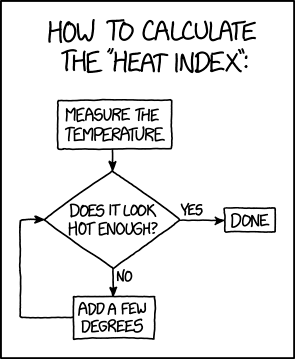Temperatures taken from this website:
https://www.historique-meteo.net/france/rh-ne-alpes/lyon/

This dataset is updated monthly to be updated early september with august temeratures (to be updated in early september with august temeratures).

python import numpy as np import pandas as pd import matplotlib.pyplot as plt plt.style.use('ggplot') %matplotlib inline

## Import and inspect the data

python

# url = 'https://www.historique-meteo.net/site/export.php?ville_id=201'

df = pd.readcsv('./data/export-lyon.csv', header=3, parsedates=['DATE'], index_col='DATE') df.info() 

<class 'pandas.core.frame.DataFrame'>
DatetimeIndex: 3499 entries, 2009-01-01 to 2018-07-31
Data columns (total 17 columns):
MAX_TEMPERATURE_C         3499 non-null int64
MIN_TEMPERATURE_C         3499 non-null int64
WINDSPEED_MAX_KMH         3499 non-null int64
TEMPERATURE_MORNING_C     3499 non-null int64
TEMPERATURE_NOON_C        3499 non-null int64
TEMPERATURE_EVENING_C     3499 non-null int64
PRECIP_TOTAL_DAY_MM       3499 non-null float64
HUMIDITY_MAX_PERCENT      3499 non-null int64
VISIBILITY_AVG_KM         3499 non-null float64
PRESSURE_MAX_MB           3499 non-null int64
CLOUDCOVER_AVG_PERCENT    3499 non-null float64
HEATINDEX_MAX_C           3499 non-null int64
DEWPOINT_MAX_C            3499 non-null int64
WINDTEMP_MAX_C            3499 non-null int64
WEATHER_CODE_MORNING      3499 non-null int64
WEATHER_CODE_NOON         3499 non-null int64
WEATHER_CODE_EVENING      3499 non-null int64
dtypes: float64(3), int64(14)
memory usage: 492.0 KB
 

What we are most interested in are min/max temperatures:

python n_days = 15 win_type = None df[['MAX_TEMPERATURE_C', 'MIN_TEMPERATURE_C']].rolling(window=n_days, win_type=win_type).mean().plot(figsize=(14, 7))

<matplotlib.axes._subplots.AxesSubplot at 0x7fd309550908>## Heat wave and warm day definitions

Meteorological services warn that there is a risk of heatwave when for at least three days, minimum temperatures, especially at night, are above 20 °C and maximum temperatures above 33 °C.

From here (in french).

So let's define a warm day to have MAX_TEMPERATURE_C >= 33 and MIN_TEMPERATURE_C >= 20. A heat wave is made of at least 3 consecutive warm days.

## Number of warm days per year

python df['WARM_DAY'] = False df.loc[(df.MAX_TEMPERATURE_C >= 33) & (df.MIN_TEMPERATURE_C >= 20), 'WARM_DAY'] = True

python print(len(df[df.WARM_DAY]), 'warm days between', df.index.date(), 'and', df.index[-1].date())

97 warm days between 2009-01-01 and 2018-07-31
 

python yearly_count = df['WARM_DAY'].resample('Y').sum().to_frame() yearly_count['year'] = yearly_count.index.year yearly_count.set_index('year', inplace=True) yearly_count.plot.bar(figsize=(14, 7), legend=False) plt.xlabel("Year") plt.ylabel("Number of warm days per year")

Text(0,0.5,'Number of warm days per year')## Number of high heat index days per year ("apparent temperature")

Effects of the heat index from wikipedia:

| Heat Index | Effect | |--- | --- | | 27–32 °C | Caution: fatigue is possible with prolonged exposure and activity. Continuing activity could result in heat cramps. | | 32–41 °C | Extreme caution: heat cramps and heat exhaustion are possible. Continuing activity could result in heat stroke. | | 41–54 °C | Danger: heat cramps and heat exhaustion are likely; heat stroke is probable with continued activity. | | over 54 °C | Extreme danger: heat stroke is imminent. |

python df['WARM_DAY_HI_27-31'] = False df['WARM_DAY_HI_32-40'] = False df['WARM_DAY_HI_over_41'] = False df.loc[(df.HEATINDEX_MAX_C >= 27) & (df.HEATINDEX_MAX_C < 32), 'WARM_DAY_HI_27-31'] = True df.loc[(df.HEATINDEX_MAX_C >= 32) & (df.HEATINDEX_MAX_C < 41), 'WARM_DAY_HI_32-40'] = True df.loc[df.HEATINDEX_MAX_C >= 41, 'WARM_DAY_HI_over_41'] = True

python yearly_count_HI = df[['WARM_DAY_HI_27-31', 'WARM_DAY_HI_32-40', 'WARM_DAY_HI_over_41']].resample('Y').sum() yearly_count_HI['year'] = yearly_count_HI.index.year yearly_count_HI.set_index('year', inplace=True)

python renaming = {'WARM_DAY': 'warm day count', 'WARM_DAY_HI_27-31': 'days with H.I. in [27, 32)', 'WARM_DAY_HI_32-40': 'days with H.I. in [32, 41)', 'WARM_DAY_HI_over_41': 'days with H.I. over 41'} pd.merge(yearly_count, yearly_count_HI, on='year').rename(renaming, axis=1).plot.bar(figsize=(14, 7))

<matplotlib.axes._subplots.AxesSubplot at 0x7fd30584bf98>It is rather difficult to use the heat index because it may differ a lot from the temperature (https://xkcd.com/2026/). Let us stick to the min/max temperatures.## Longest heat wave per year

python def countcontiguouswarm_days(vec):

CONT_WARM_DAYS = np.zeros(vec.shape, dtype=int)
count = 0
for i in range(1, vec.shape):
if vec[i] == 1:
count += 1
CONT_WARM_DAYS[i] = count
else:
count = 0
for i in range(vec.shape-2, -1, -1):
if CONT_WARM_DAYS[i] > 0:
if CONT_WARM_DAYS[i+1] > 0:
CONT_WARM_DAYS[i] = CONT_WARM_DAYS[i+1]

return CONT_WARM_DAYS
 



python df['CONT_WARM_DAYS'] = count_contiguous_warm_days(df.WARM_DAY.values)

python longest_heatwave = df['CONT_WARM_DAYS'].resample('Y').max().fillna(0).to_frame() longest_heatwave['year'] = longest_heatwave.index.year longest_heatwave.set_index('year', inplace=True)

python longest_heatwave.sort_values('CONT_WARM_DAYS', ascending=False)[:5].plot.bar(figsize=(10, 5), legend=False) plt.xlabel("Top 5 years") plt.ylabel("Longest heat wave per year (days)")

Text(0,0.5,'Longest heat wave per year (days)')## Cumulative number of heat wave days per year

Here we only consider days in heat waves (with at least 3 consecutive warm days).

python title = 'Cumulative number of heat wave days' heatwave_days = df[df.CONT_WARM_DAYS >= 3].CONT_WARM_DAYS.resample('Y').count() heatwave_days.name = title heatwave_days = heatwave_days.to_frame() heatwave_days['year'] = heatwave_days.index.year heatwave_days.set_index('year', inplace=True)

python heatwave_days.sort_values(title, ascending=False)[:5].plot.bar(figsize=(10, 5), legend=False) plt.xlabel("Top 5 years") plt.ylabel(title)

Text(0,0.5,'Cumulative number of heat wave days')## Peak temperature

python yearly_peak = df['MAX_TEMPERATURE_C'].resample('Y').max().to_frame() yearly_peak['year'] = yearly_peak.index.year yearly_peak.set_index('year', inplace=True)

python yearly_peak.sort_values("MAX_TEMPERATURE_C", ascending=False)[:5].plot.bar(figsize=(10, 5), legend=False) plt.xlabel("Top 5 years") plt.ylabel("Peak temperature")

Text(0,0.5,'Peak temperature')## Average temperature in June, July and August

The formula used to compute the monthly temperature is not very sophisticated... We take the mean of the min and max temperature over the month.

python df_month = df[['MAX_TEMPERATURE_C', 'MIN_TEMPERATURE_C']].resample('M').mean() df_month['MEAN_TEMP'] = df_month.mean(axis=1) df_month['year'] = df_month.index.year df_month['month'] = df_month.index.month

python df_june = df_month[df_month.month==6][['year', 'MEAN_TEMP']] df_july = df_month[df_month.month==7][['year', 'MEAN_TEMP']] df_august = df_month[df_month.month==8][['year', 'MEAN_TEMP']]

python df_summer_months = pd.DataFrame({'june': df_june.MEAN_TEMP.values}, index=df_june.year.values) df_summer_months = df_summer_months.join(df_july.rename({'MEAN_TEMP': 'july'}, axis=1).set_index('year')) df_summer_months = df_summer_months.join(df_august.rename({'MEAN_TEMP': 'august'}, axis=1).set_index('year')) df_summer_months.plot.bar(figsize=(14, 7)) plt.xlabel("Year") plt.ylabel("Mean temperature of the summer months")

Text(0,0.5,'Mean temperature of the summer months')## Conclusion

This month of July was hot...